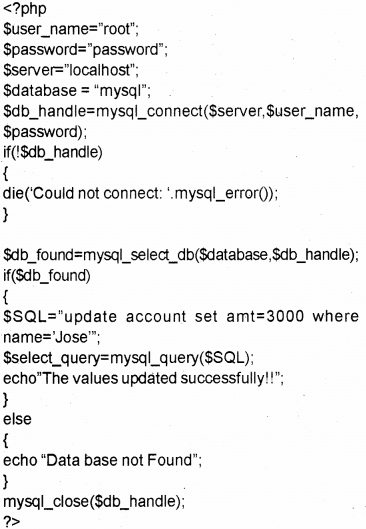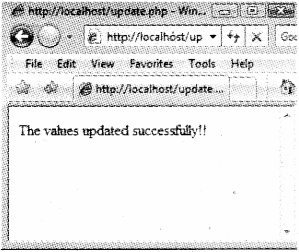# Plus Two Computer Science Notes Chapter 10 Server Side Scripting Using PHP

Kerala State Board New Syllabus Plus Two Computer Science Notes Chapter 10 Server Side Scripting Using PHP.

## Kerala Plus Two Computer Science Notes Chapter 10 Server Side Scripting Using PHP

PHP stands for PHP Hypertext Preprocessor. Normally, it runs on a web server.

Benefits of using PHP

Client-side scripts(JavaScript) are run faster but it has some limitations. The limitations are we can view the source code hence it is less secure. On the other hand PHP is executed on the server and the result is sent back to the client(browser) so it is impossible to view the source code.

Benefits of PHP are given below

• As PHP can be easily embedded into HTML moreover its easiness to convert a static web site into dynamic one.
• It is compatible with almost all databases
• It is platform independent can run on almost all OS (Windows, Linux, MAC)
• It is simple and easy to learn
• It uses very small amount of system resources
• to run and faster.
• PHP provides security to prevent malicious attacks.
• Open source community supports PHP
• It is a free s/w by all means hence can be used to develop all types of web sites

Basics of PHP

A) Setting up the development environment

Step 1

• Install a PHP compatible web Server (Eg. Abyss Web Server For Windows)

Step 2

• Install PHP interpreter.
After installing the web server type http://localhost in the address bar the following screen will be available.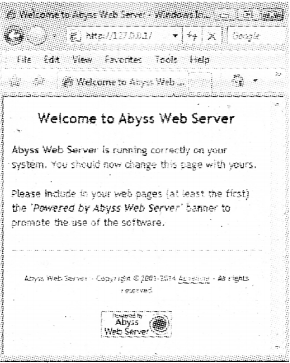B) Writing and running the script

Take a note pad and type the code, PHP code should begin with <?php andend with ?>. Save this file in the root directory of the web server with extension php.

Step 1
Take a notepad and type (he following and save it as first.php on C:\Abyss Web ServeAhtdocs.
<?php
echO”My first PHP web page”;
?>

Step 2
Start the web server if it is off

Step 3
Type as “http://localhost/firstphp” in the address bar.C) Combining HTML and PHP
Both are closely related. We can write PHP code in between HTML code using <?php and ?>.
Eg: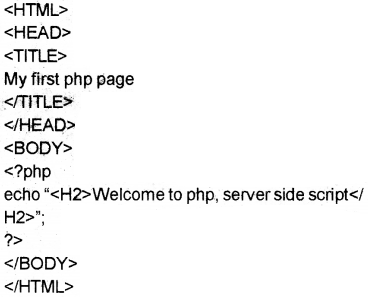We can make some text as a comment i.e. not as part of the program Single line(//) and multi line (starts with /* and ends with */)

<HTML>
<TITLE>
My first php page </TITLE>
<BODY>
<?php
/* This program is used to display a message “Welcome to php” */ echo “<H2>Welcome to php</H2>”; //shows a message?>
</BOD Y>
</HTML>

E) Output statements in PHP
i) echo and print are used to display all types of data but echo is used to produce multiple outputs. Parenthesis is optional,

eg:
echo “ first output”, “second output”;
or
echo (“ first output”, “second output”);
print “only one output”;
or
print (“only one output”);
The difference between echo and print

 Echo Print Take more than one parameter Only one parameter Does not return any value Returns TRUE or l on successful print and FALSE otherwise Faster slower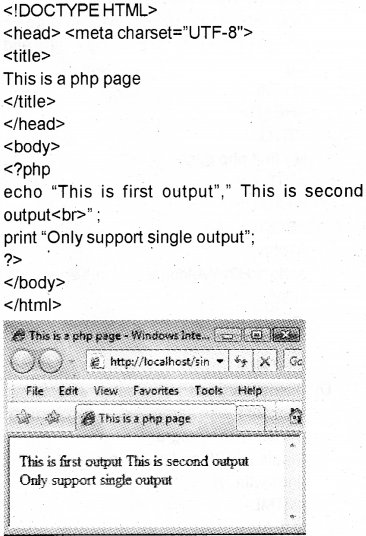ii) var_dump()
This is used to display both data type and value of variables.
Syntax: var_dumpl(variablel, vanable2,etc…);

Eg: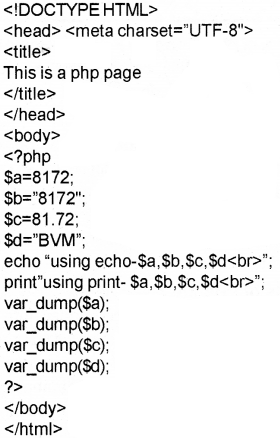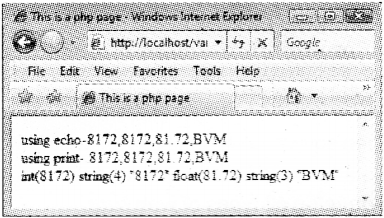Fundamentals of PHP

Variables

A variable name starts with $symbol followed by the name of the variable. There is no need to declare a variable before using it. PHP automatically determines the data type based upon the value stored in it. Syntax:$variable_name=value;

Rules for naming a variable in PHP

• Begins with $symbol followed by an alphabet or under score(a-zA-Zand _) • Digits(O-9)can be used after 2 characters.(Eg.$_1 ,$al ,$s12,etc)
• Variable names are case sensitive.
• Special characters cannot used except under score.

Data type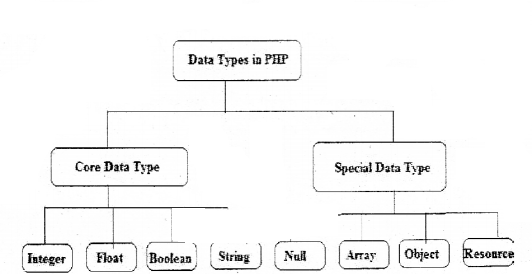A) Core data types
Integer, Float/ Double and String, these data types are similar data types of C++. The size of integer or float is depending upon the OS.

• Integer—Two types—ye and +ve.. PHPdoesnot support unsigned integer data type.
Eg: 8172, -22, etc.
• Float/ Double -:Numbers with decimal point.
Eg: 81.72, 7E-1 0(7 * 1010 —Mantissa and Exponent method), 123E1 2(123*1012)
• String: Combination of characters enclosed in quotes(single or double quotes) Eg: “BVM”,’HSS’, Computer Science’, etc.
• Boolean: Used to store two values(states) TRUE or FALSE.
Eg: values are yes/no, on/off, 1/0, true/false
<?php
Sx=false; Il assign the value FALSE to $x.$y=1; //assign the value TRUE to $y. B) Special data types • Null-: Specialdata type with value NULL. This is the default value to a variable, if we do not set a value to it.$rn=null;
• Array -: Array is a collection of elements. With the same name we can store many elements.
Three types of array
Indexed array-:
Associated array-:
Multi dimensional array-:
• Object -; Similar in C++ and must be declared explicitly. At first a class is declared and then objects can be created using the command new.
• Resources -: It is not an actual data type rather it is a special variable that hold references to file handler, data base object, etc.

Operators in PHP

Operators are the symbols used to perform an operation
a) Assignment operator(=)
The value of RHS is assigned to LHS variable. If $a=10 and$b=3 then $a=$b. This statement sets the value of $a and$b are same ,i.e. it sets a to 3.

b) Arithmetic operators
It is a binary operator. It is used to perform addition (+), subtraction(-), division(/), multiplication(*) ,modulus(%-givesthe remainder).
Eg. If $x=10 and$y=3then

 $x+$y $x-$y $x*$y $x/$y $x%$y 13 7 30 3.333 1

c) Relational (Comparison) operators
It is used to perform comparison or relational operation between two values and returns either true or false.
Eg: If $X=10and$Y=3then

 $X<$Y $X<=$Y $X>$Y $X>=$Y $X>==$Y $X!=$Y false false true true false true

d) Logical operators
Here (and , &&), (or, ||), xorare binary operators and not(!) is a unary operator. It is used to combine relational operations and it gives either true or false
If $X=true and$Y=false then

 $x &&$x $X &&$Y $Y &&$X $Y &&$Y true false False false

Both operands must be true to get a true value in the case of && operation If $X=true and$Y=false then

 $X and$X $X and$Y $Y and$X $Y and$Y true false False false

Both operands must be true to get a true value in the case of ‘and’ operation If $X=true and$Y=false then

 $X ||$X $X ||$Y SY || $X$Y || $Y true true true false Either one of the operands must be true to get a true value in the case of || operation If$X=true and $Y=false then $X or $X$X or $Y$Y or $X$Y or $Y true true true false Either one of the operands must be true to get a true value in the case of or operation If SX=true and$Y=false then

 $X xor$X $X xor$Y $Y xor$X $Y xor$Y true true true false

Either one of the operands must be true to get a true value in the case of xor operation If $X= true and$Y=false then

 l$X !$Y false true

This is also called concatenation operator. It joins(concatenates) two strings and forms a string.
They are and ‘.=’.
Eg:
$x= “BVM HSS$y= “ Kalparamba”;
$z=$x.$y; • Here the variable z becomes “BVM HSS Kalparamba”.$x.=$y; • Here the variable$x becomes “BVM HSS Kalparamba”.

f) Combined operators.
It is also called short hands
If $X=1O and$Y= 3 then

 Arithmetic Assignment Expression Equivalent Arithmetic Expression The value of $X becomes$X+=$Y$X=$X+$Y 13 $X-=$Y $X=$X-$Y 7$X*=$Y$X=$X*$Y 30 $X/=$Y $X=$X/$Y 3.333$X%=$Y$X=$X%$Y 1

g) Increment and decrement operators.
1) pre increment (++variable)
Eg:
lf$x=10then echo(++$x); -> It prints 10+1=11

2) post increment (variable++)
lf $x=10then echo($x++); -> It prints 10 itself.

3) pre decrement—variable)
lf $x=10then echo(—$x); It prints 10-1=9

4) post decrement(variable—)
lf $x=10then echo($x—);-> It prints 10 itself.

h) Escape sequences
It Is a character preceded by a back slash(\)

 Operator Definition Example Output \“ To print” echo TPHPr “PHP’ \’ To print1 echo”X\ian” X’ian \n New line echo “BVM \nHSS” BVM HSS \t To print tab echo”BVM\tHSS” BVM HSS \r Print carriage return \$To prints echo”100\$” 100$\\ To print \ echo”\\n is used as newline” \n is used as new line Control Structures in PHP In general the execution of the program is sequential, we can change the normal execution by using the control structures. simple if Syntax:The first expression evaluated and selects the statements with matched case value. Loops in PHP a) while loop It is an entry controlled loop The syntax is given below Loop variable initialised while(expression) { Body of the loop; Update loop variable; } b) do-while loop This loop will execute at least once. The body of the loop will be executed until the expression becomes false, do { Body of the loop; Update loop variable; }while(expression); * for loop The syntax of for loop is given below For(initialisation; testing; updation) { Body of the for loop; } continue and break in loops Continue is used to skip one iteration and continue the loop with next value. But break is used to terminate the execution of the current control structure such as for, for each, while, do while or switch. Arrays in PHP In PHP array is a collection of key and value pairs. Unlike C++, in PHP we can use either non negative integers or strings as keys. Different types of arrays in PHP • Indexed arrays • Associate arrays • Multi dimensional arrays. Indexed arrays-: The elements in an array can be distinguished as first or second or third etc. by the indices and the index of the first element is zero. In PHPthe function arrayO is used to create an array. Syntax:$array_name=array(value1 ,value2, );
OR
$array_variable[key]=value; Eg:$mark=array(60,70,80);
$course=amay(“Science”,”Commence”,”Humanities’); OR$mark=60;
$mark=70;$mark=80;
$course=”Science”;$course=”Commerce”;
$course=”Humanities”; Eg: <!DOCTYPE HTML> <html lang=”eii”> <head> <title> We are learning PHP </title> </head> <body bgcoior=”cyan”> <?php$course=amay(“Science”, “Commerce”,”Humanities’);
for($i=0;$i<3;$i++) echo$course[$i].”,”; ?> </body> </html>The function print_r can be used for printing an array. Syntax: print_r(array_name); Eg:Associative arrays Arrays with named keys and string indices are called associative jarrays. Syntax:$varibale_name=array(key1=>value1, key2=>value2,etc);

Eg: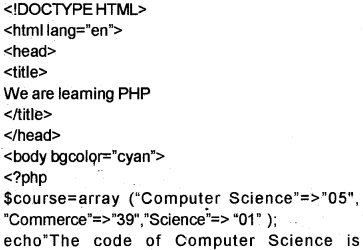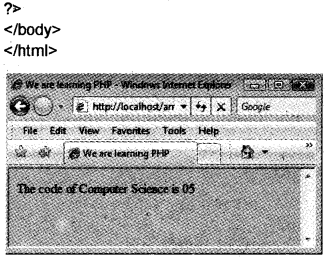for each loops (Extension of for loop)

The number of elements in an array is not known in advance foreach loop is used. Two types

1) for ($array_variable_name as$value)
No need to specify the starting inde and ending index, the array pointer is moved one by one.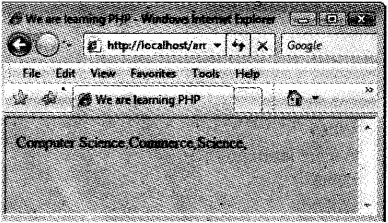b) foreach($array_variable_name as key=>$value)
Here the value of the current array element is assigned to $value and the key is assigned to$key.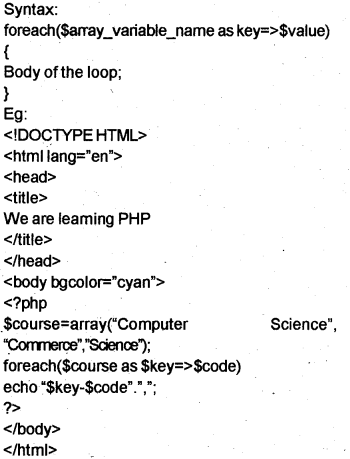FunctionsinPHP

Two types user defined and built in functions.

User-defined functions

The key word function is used to declare a function in PHP.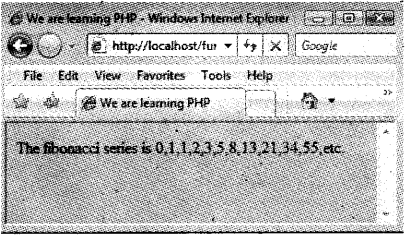Built-in functions

a) Date() -:Returns date and time.
Syntax: date(format[, timestamp]);

 Format Purpose d day of the month(0-31) m month(1-12) Y year I day of the week(Eg.Monday)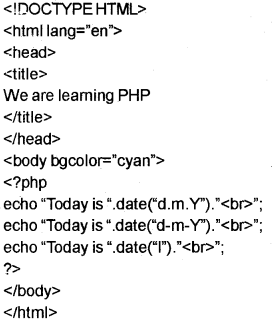b) String functions

• chr()-: Returns a character corresponding to the ASCI I. Eg. chr(ascii value);
• strlen()- Returns the number of characters in a string strlen(string);
• strops()- Returns the position of the first occurrence of a string inside another string. strpos(main string, substring, start position);
• strcmp()- Compares two stings, strcm p(stri ng 1 ,stri ng2);Three tier architecture in PHP

Tier 1 (Front end Tool): Browser/ Client uses HTML or HTML embedded with JavaScript.
Tier 2 (Middle end Tool)- Web Server(Apache /Microsoft IIS)
Tier 3 (Back end Tool)-Database(MySQL/Oracle) This means end user uses Browser(Tier 1) and send requests to the Web Server(Tier 2). The Web Server retrieves data from the Data Base(Tier 3). For this activities user interface, application programs, data storage etc are separated into layers(Tiers)

PHP forms

This is used to collect information from the client and send it to the Web Server by pressing the submit button. The server receives the data and collects the appropriate data from the data base and send it back as a response.

PHP global variables

A variable declared as global then it can be accessed from any where in the program.

• Superglobal arrays : This is a special variable – available to all programs.
 Superglobal Purpose SGLOBALS Global scope variables available. . $_SERVER Information about the server$_GET Data passed using the HTTP GET method $_POST Data passed using the HTTP POST method$_REQUEST Data passed via HTTP request $_FILES Data passed by an HTML file input$_SESSION Current session data specific to the user $_COOKIE Data stored on the browser. A) PHP$GLOBALS
PHP stores all global variables in an arräy calied $GLOBALS[index]. Index is the name of the variable.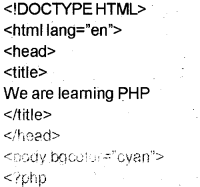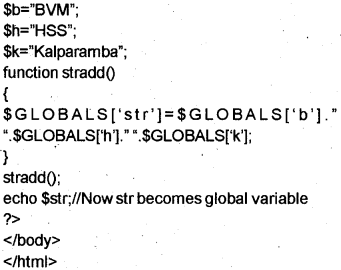B) PHP$_SERVER
This returns the paths and script locations.

 Code Description $_SERVER [‘PHP_SELF] Returns the currently executing file name$_SERVER [‘SERVER_NAME’] Returns the host server name $_SERVER [‘SCRIPT_NAME’] same as$_SERVER[‘PHP_SELF’]C) PHP $_REQUEST When a user click a submit button,$_REQUEST gathers all data. $_REQUEST is an array that contains$_GET, $_POST and$_cookie superglobals.

D) PHP $_POST When the user presses the submit button and the method=post then the data is passed to the server and we can access the data by using the variable$_POST[‘variable name’];

E) PHP S_GET
When the user presses the submit button and the method=get then the data is passed to the seÑer and we can access the data by using the variable $_GET[’vanable namel; GET and POST comparison The difference between GET and POST method is given below.  Get method Post method 1. Faster 1. Slower 2. To send small volume of data. 2. To send large volume of data 3. Up to 2000 characters 3. No limit 4. Less secure 4. More secure 5. Data visible during submission 5. Data not visible during submission 6. Page link can be book marked 6. Page link can never be book marked. Connecting PHP to database(MySQL) Establishing connection to MySQL database To access data from the data base, the PHP program first establish a connection to the data base server(MySQL) and then specify the data base to use.Consider the following • Open a connection to MySQL. • Secify the data base we want to open. • Retrieve data from or insert data in to dat base. • Close the connection. Step 1 Open a connection to MySQL For this rnysql_connect function is used. A link identifier is returned when connected successfully otherwise a NULL value.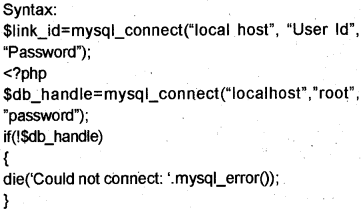echo connected Successfully’; mysql_dose($dbhandle);
?>

Note : In the above specify the password you given in MVSQL installation.

The out put is as given below.Step 2
Specify the data base you want to open
MySQL server may contain many data bases. mysql_select_db() is a function used to select particular data base. It return true if it finds the data base otherwise false.
Syntax: mysql_select_db($database); Eg: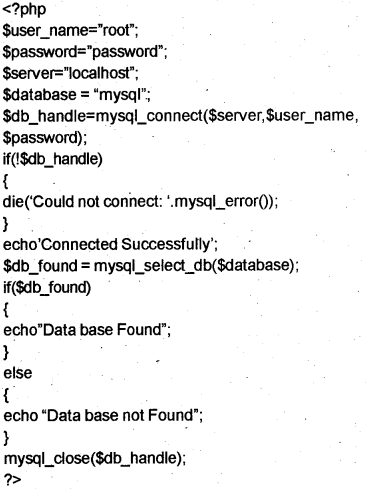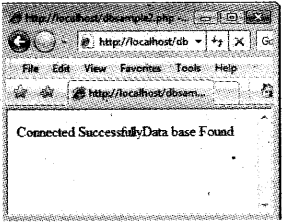Step 3 Reading data from data base Two steps a) Execute the SQL query on the data base using the function mysql_query() Syntax:$result_set=mysql_query(query,connection);

b) Insert a row using the function mysql_fetch_array()
Sresult_set=mysql_query(query, connection);
$fetched_row=mysql_fetch_array($result_set);

Example 1 :
Let us do the following program The structure of a table is given to store the details of marks scored by students in an exam

 Data Type Description RegNo Numeric Unique name char upto 30 characters course char marks of 6 subjects numeric six separate columns

Write SQL statements for the creation of the table and the following requirements.

Step 1.
For this take MySQL and do the following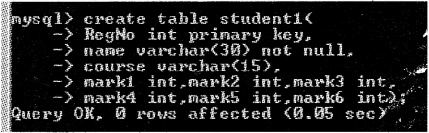a) Insert data into the fields(min 3 records)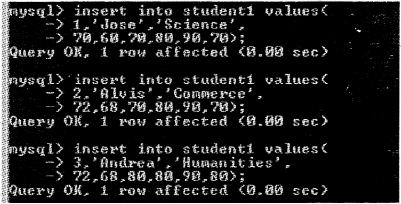b) Display the details of all students.Step 2:
Take a notepad and type the following then save it asform1.html

<html>
<body>
<form method-’post” action=”dbsample3.php”>
<center>RegisterNo:
<inputtype=”text” name=”regno”><br>
<input type=”submit” value=”Show Result”>
</center>
</form>
</body>
</html>

Step 3.
Take another notepad then type the following and save itasdbsampleS.php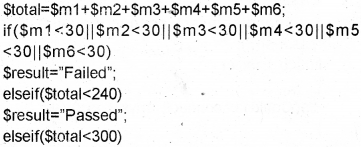Step 4.
Run the file forml .html and do the following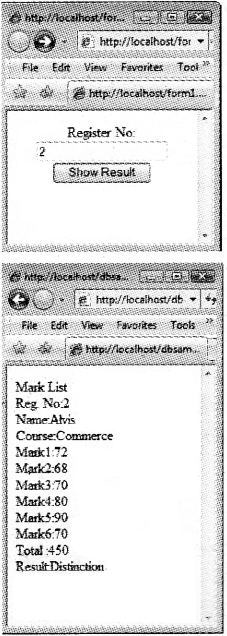Example 2

Step 1.
Take a notepad and type the following. Save it as form2.html.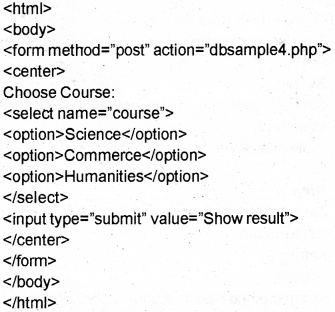Step 2
Take another notepad then type the following and save it as dbsample4.php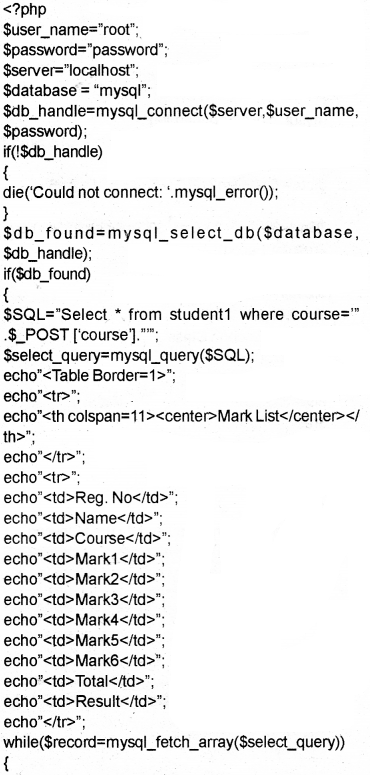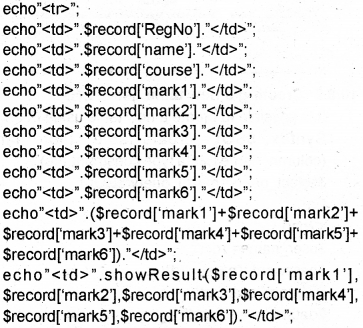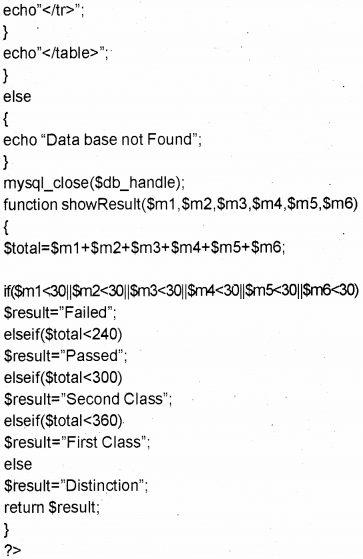Step 3.
Run the file form2.html and do the following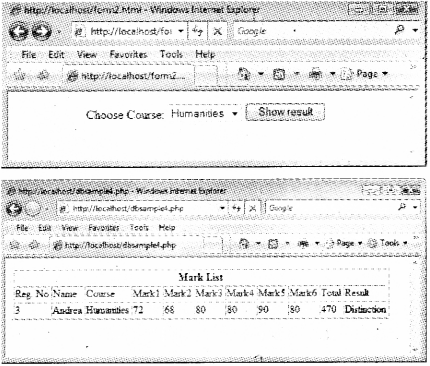Creating a new table using PHP

It is possible to create a new table using PHP.
Syntax: $SQL=”create table <table name> (column_name1 data type constraint,etc)”;$select_query=mysql_query($SQL); Eg: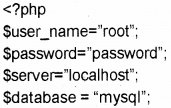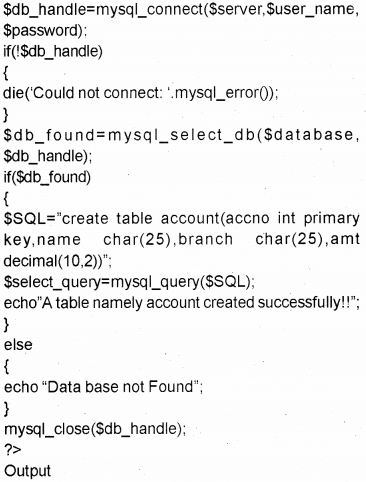Inserting data into a table using PHP It is also possible to insert values in to a table using PHP program Syntax:$SQL=”insert into <table name> values (valuel, value2,…);
$select_query=mysql_query($SQL);

Ex:
Step 1.
Take a notepad .then type the following and save it asform3.html
<html>
<body>
<form method-’post” action-’table2.php”>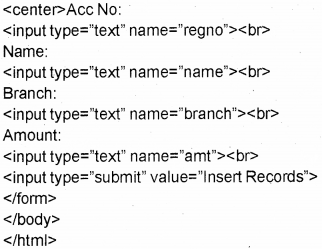Step 2.
Take another notepad and type the following then save it as table2.php.Step 3.
Run the file form3.html and do the followingUpdating data in a table using PHP

It is also possible to change or update values in a row by using PHP.
$SQL=”Update ctable_name> set ccolumn_name>= new_value where condition.$select_query=mysql_query(\$SQL);

Ex:
If you want to change the amount deposited by Jose from 2000 to 3000. Do the following.

Step 1.
Take a notepad, then type the following and save it asform3.html